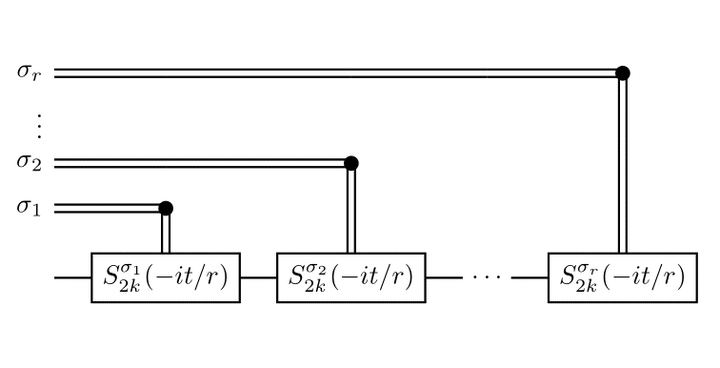# Faster quantum simulation by randomization### Abstract

Product formulas can be used to simulate Hamiltonian dynamics on a quantum computer by approximating the exponential of a sum of operators by a product of exponentials of the individual summands. This approach is both straightforward and surprisingly efficient. We show that by simply randomizing how the summands are ordered, one can prove stronger bounds on the quality of approximation for product formulas of any given order, and thereby give more efficient simulations. Indeed, we show that these bounds can be asymptotically better than previous bounds that exploit commutation between the summands, despite using much less information about the structure of the Hamiltonian. Numerical evidence suggests that the randomized approach has better empirical performance as well.

Type
Publication
Quantum 3, 182 (2019)##### Yuan Su
###### Research Scientist

I work on quantum algorithms for simulating Hamiltonian dynamics. I am particularly interested in the design, analysis, implementation, and application of quantum simulation.